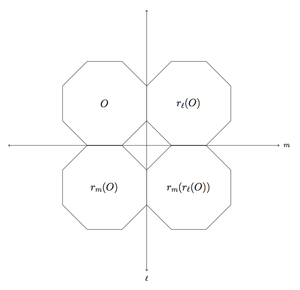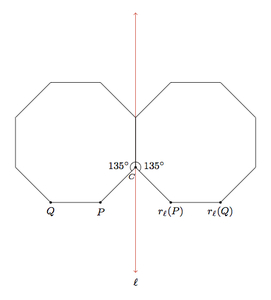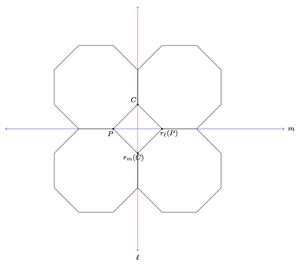# Building a tile pattern by reflecting octagons

Alignments to Content Standards: G-CO.B.6

Below is a picture of a regular octagon, which we denote by $O$, and two lines denoted $\ell$ and $m$, each containing one side of the octagon:1. Draw $r_\ell(O)$, the reflection of the octagon about $\ell$.
2. Draw $r_m(O)$ and $r_m(r_\ell(O))$, the reflections of the two octagons from part (a) about line $m$.
3. Show that the quadrilateral enclosed by the four octagons $O$, $r_\ell(O)$, $r_m(O)$, and $r_m(r_\ell(O))$ found in parts (a) and (b) is a square.

## IM Commentary

This task applies reflections to a regular octagon to construct a pattern of four octagons enclosing a quadrilateral: the focus of the task is on using the properties of reflections to deduce that the quadrilateral is actually a square. This pattern is also studied in 8.G Tile Patterns I: octagons and squares where the four octagons are given from the outset rather than being developed using reflections.

This task uses the fact that the interior angles of a regular octagon measure $135^\circ$: this can be shown as in 8.G Tile Patterns I: octagons and squares or using the methods of A-CED Sum of angles in a polygon. Students can also deduce this as part of the problem but this calculation is secondary to careful reasoning about reflections.

The line $\ell$ contains one side of the given octagon $O$ and it also contains the reflection of that side on $r_\ell(O)$. Students may take this fact for granted and the teacher will need to decide whether or not it is important to pursue an explanation as provided in the solution. This depends on the students: if they are just gaining familiarity with applying reflections then this can be passed over. If they are working explicitly with the formal definition of reflections, however, then this is a good opportunity to apply this definition.

The pattern of octagons surrounding a square can be extended indefinitely in both horizontal and vertical directions: reflecting about the left or right vertical edge of the figure extends the pattern in the horizontal direction while reflecting about the upper or lower horizontal edge of the figure extends it in a vertical direction. Repeated reflections generate the pattern seen here:

Finally, we mention that variants of the solution below can be found, which may be more in line with an teacher's prior instruction. For example, students could recognize that since each of the sides of the quadrilateral is constructed via a series of reflections from the original side, they are all congruent to one another. From here, it suffices to observe that even just one of the angles of the quadrilateral is a right angle, to prove that the quadrilateral is a square.

## Solution

1. Below is a picture of the octagon along with the reflection of the octagon about $\ell$:The picture will be discussed and explained in part (c) below.

2. Below is a picture of the two octagons from part (a) and their reflections about $m$:This picture will also be discussed and explained in part (c) below.

3. To justify the pictures in parts (a) and (b) and explain why the inner quadrilateral bounded by the four octagons is a square, we begin by studying the reflection of $O$ about $\ell$ more closely. Below is a picture with two vertices of $O$, and their reflections about $\ell$, labelled:Angle $C$ forms a complete circle together with the two interior angles marked $135^\circ$. We know that these angles are congruent because reflections preserve angle measures. We know that they must both measure $135^\circ$ because the interior angles of the regular octagon $O$ each measure $135^\circ$. There are $360$ degrees in a circle so this means that $m(\angle C) = 90$.

We claim next that $Q,P,r(P),$ and $r(Q)$ are collinear. From the definition of reflections, $\ell$ is the perpendicular bisector of the segments $\overline{Pr_\ell(P)}$ and $\overline{Qr_\ell(Q)}$ so $Q,P,r_\ell(P),$ and $r_\ell(Q)$ will all be collinear provided line $\overline{PQ}$ is perpendicular to line $\ell$. Below is a picture of the point $S$ where line $\overleftrightarrow{PQ}$ meets $\ell$:We know from above that $m(\angle SPC) = m(\angle SCP) = 45$ since these two angles are each supplementary with an interior angle of a regular octagon which measures $135^\circ$. Since the sum of the angles in triangle $SPC$ is $180^\circ$ this means that angle $CSP$ is a right angle. So line $\overleftrightarrow{PQ}$ is perpendicular to $\ell$ and so $r_\ell(P)$ and $r_\ell(Q)$ are collinear with $P$ and $Q$ as desired.

In part (b) of the problem we reflect the two octagons above about line $m$. This is pictured below, with the point $C$ discussed above and its reflection about $m$ plotted:All four sides of quadrilateral $PCr_\ell(P)r_m(C)$ are congruent to segment $\overline{PC}$ because they are obtained by reflecting this segment over $\ell$, $m$, $\ell$ and then $m$. Angle $C$ has been shown to be a right angle and so angle $r_m(C)$ is also a right angle because reflections preserve angles. Finally, angles $CPr_m(C)$ and $Cr_\ell(P)r_m(C)$ are right angles because they are bisected by line $m$ (since reflection about $m$ preserves angle measurements) and angles $CPr_\ell(P)$ and $Cr_\ell(P)P$ are both $45^\circ$ angles. So quadrilateral $PCr_\ell(P)r_m(C)$ is a square since it has four right angles and four congruent sides.